# NCERT solutions for Class 9 Maths chapter 8 - Quadrilaterals [Latest edition]

#### Chapters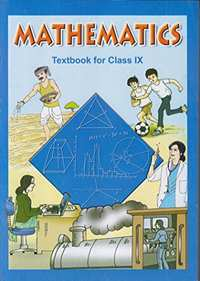## Solutions for Chapter 8: Quadrilaterals

Below listed, you can find solutions for Chapter 8 of CBSE NCERT for Class 9 Maths.

Exercise 8.1Exercise 8.2
Exercise 8.1 [Pages 146 - 147]

### NCERT solutions for Class 9 Maths Chapter 8 Quadrilaterals Exercise 8.1 [Pages 146 - 147]

Exercise 8.1 | Q 1 | Page 146

The angles of quadrilateral are in the ratio 3: 5: 9: 13. Find all the angles of the quadrilateral.

Exercise 8.1 | Q 2 | Page 146

If the diagonals of a parallelogram are equal, then show that it is a rectangle.

Exercise 8.1 | Q 3 | Page 146

Show that if the diagonals of a quadrilateral bisect each other at right angles, then it is a rhombus.

Exercise 8.1 | Q 4 | Page 146

Show that the diagonals of a square are equal and bisect each other at right angles.

Exercise 8.1 | Q 5 | Page 146

Show that if the diagonals of a quadrilateral are equal and bisect each other at right angles, then it is a square.

Exercise 8.1 | Q 6 | Page 146

Diagonal AC of a parallelogram ABCD bisects ∠A (see the given figure). Show that

(i) It bisects ∠C also,

(ii) ABCD is a rhombus.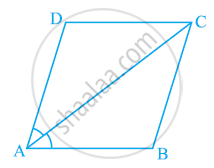Exercise 8.1 | Q 7 | Page 146

ABCD is a rhombus. Show that diagonal AC bisects ∠A as well as ∠C and diagonal BD bisects ∠B as well as ∠D.

Exercise 8.1 | Q 8 | Page 146

ABCD is a rectangle in which diagonal AC bisects ∠A as well as ∠C. Show that:-

(i) ABCD is a square (ii) diagonal BD bisects ∠B as well as ∠D.

Exercise 8.1 | Q 9 | Page 147

In parallelogram ABCD, two points P and Q are taken on diagonal BD such that DP = BQ (see the given figure). Show that:

(i) ΔAPD ≅ ΔCQB

(ii) AP = CQ

(iii) ΔAQB ≅ ΔCPD

(iv) AQ = CP

(v) APCQ is a parallelogram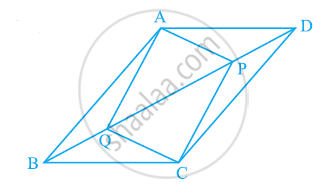Exercise 8.1 | Q 10 | Page 147

ABCD is a parallelogram and AP and CQ are perpendiculars from vertices A and C on diagonal BD (See the given figure). Show that

(i) ΔAPB ≅ ΔCQD

(ii) AP = CQ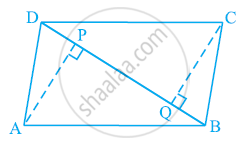Exercise 8.1 | Q 11 | Page 147

In ΔABC and ΔDEF, AB = DE, AB || DE, BC = EF and BC || EF. Vertices A, B and C are joined to vertices D, E and F respectively (see the given figure). Show that

(i) Quadrilateral ABED is a parallelogram

(ii) Quadrilateral BEFC is a parallelogram

(iv) Quadrilateral ACFD is a parallelogram

(v) AC = DF

(vi) ΔABC ≅ ΔDEF.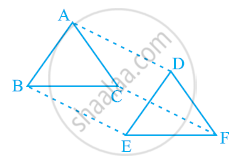Exercise 8.1 | Q 12 | Page 147

ABCD is a trapezium in which AB || CD and AD = BC (see the given figure). Show that

(i) ∠A = ∠B

(ii) ∠C = ∠D

(iv) diagonal AC = diagonal BD

[Hint: Extend AB and draw a line through C parallel to DA intersecting AB produced at E.]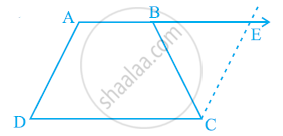Exercise 8.2 [Pages 150 - 151]

### NCERT solutions for Class 9 Maths Chapter 8 Quadrilaterals Exercise 8.2 [Pages 150 - 151]

Exercise 8.2 | Q 1 | Page 150

ABCD is a quadrilateral in which P, Q, R and S are mid-points of the sides AB, BC, CD and DA (see the given figure). AC is a diagonal. Show that:

(i) SR || AC and SR = 1/2AC

(ii) PQ = SR

(iii) PQRS is a parallelogram.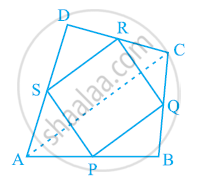Exercise 8.2 | Q 2 | Page 150

ABCD is a rhombus and P, Q, R and S are the mid-points of the sides AB, BC, CD and DA respectively. Show that the quadrilateral PQRS is a rectangle.

Exercise 8.2 | Q 3 | Page 150

ABCD is a rectangle and P, Q, R and S are mid-points of the sides AB, BC, CD and DA respectively. Show that the quadrilateral PQRS is a rhombus.

Exercise 8.2 | Q 4 | Page 150

ABCD is a trapezium in which AB || DC, BD is a diagonal and E is the mid - point of AD. A line is drawn through E parallel to AB intersecting BC at F (see the given figure). Show that F is the mid-point of BC.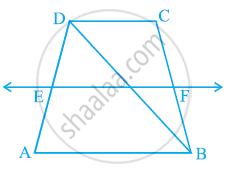Exercise 8.2 | Q 5 | Page 151

In a parallelogram ABCD, E and F are the mid-points of sides AB and CD respectively (see Fig.) Show that the line segments AF and EC trisect the diagonal BD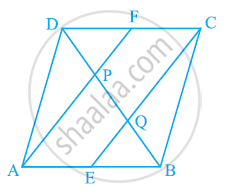Exercise 8.2 | Q 6 | Page 151

Show that the line segments joining the mid-points of the opposite sides of a quadrilateral bisect each other.

Exercise 8.2 | Q 7 | Page 151

ABC is a triangle right angled at C. A line through the mid-point M of hypotenuse AB and parallel to BC intersects AC at D. Show that

(i) D is the mid-point of AC

(ii) MD ⊥ AC

(iii) CM = MA = 1/2AB

## Solutions for Chapter 8: Quadrilaterals

Exercise 8.1Exercise 8.2## NCERT solutions for Class 9 Maths chapter 8 - Quadrilaterals

Shaalaa.com has the CBSE Mathematics Class 9 Maths CBSE solutions in a manner that help students grasp basic concepts better and faster. The detailed, step-by-step solutions will help you understand the concepts better and clarify any confusion. NCERT solutions for Mathematics Class 9 Maths CBSE 8 (Quadrilaterals) include all questions with answers and detailed explanations. This will clear students' doubts about questions and improve their application skills while preparing for board exams.

Further, we at Shaalaa.com provide such solutions so students can prepare for written exams. NCERT textbook solutions can be a core help for self-study and provide excellent self-help guidance for students.

Concepts covered in Class 9 Maths chapter 8 Quadrilaterals are Concept of Quadrilaterals - Sides, Adjacent Sides, Opposite Sides, Angle, Adjacent Angles and Opposite Angles, Angle Sum Property of a Quadrilateral, Types of Quadrilaterals, Another Condition for a Quadrilateral to Be a Parallelogram, Theorem of Midpoints of Two Sides of a Triangle, Property: The Opposite Sides of a Parallelogram Are of Equal Length., Theorem: A Diagonal of a Parallelogram Divides It into Two Congruent Triangles., Theorem : If Each Pair of Opposite Sides of a Quadrilateral is Equal, Then It is a Parallelogram., Property: The Opposite Angles of a Parallelogram Are of Equal Measure., Theorem: If in a Quadrilateral, Each Pair of Opposite Angles is Equal, Then It is a Parallelogram., Property: The diagonals of a parallelogram bisect each other. (at the point of their intersection), Theorem : If the Diagonals of a Quadrilateral Bisect Each Other, Then It is a Parallelogram.

Using NCERT Class 9 Maths solutions Quadrilaterals exercise by students is an easy way to prepare for the exams, as they involve solutions arranged chapter-wise and also page-wise. The questions involved in NCERT Solutions are essential questions that can be asked in the final exam. Maximum CBSE Class 9 Maths students prefer NCERT Textbook Solutions to score more in exams.

Get the free view of Chapter 8, Quadrilaterals Class 9 Maths additional questions for Mathematics Class 9 Maths CBSE, and you can use Shaalaa.com to keep it handy for your exam preparation.